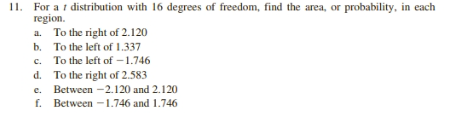# 11. For adistribution with 16 degrees of freedom, find the area, or probability, in eachregiona. To the right of 2.120To the left of 1.337b.To the left of -1.746To the right of 2.583Between -2.120 and 2.120Between 1.746 and 1.746c.d.e.f.

Question
2 viewshelp_outlineImage Transcriptionclose11. For a distribution with 16 degrees of freedom, find the area, or probability, in each region a. To the right of 2.120 To the left of 1.337 b. To the left of -1.746 To the right of 2.583 Between -2.120 and 2.120 Between 1.746 and 1.746 c. d. e. f. fullscreen
check_circle

Step 1

We are authorized to answer three subparts at a time, since you have not mentioned which part you are looking for, so we are answering the first three subparts, please repost your question separately for the remaining subpart.

Step 2

It is given that the degree of freedom is 16.

(a)

The p-value to the right of 2.120 can be calculated as:

Step 3

(b)

The p-value to the left of 1.337 ...

### Want to see the full answer?

See Solution

#### Want to see this answer and more?

Solutions are written by subject experts who are available 24/7. Questions are typically answered within 1 hour.*

See Solution
*Response times may vary by subject and question.
Tagged in

### Hypothesis Testing